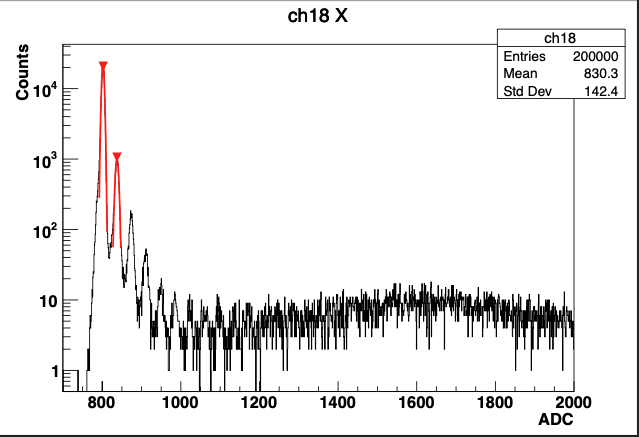# Alternative for TSpectrum?

Hi!

We are currently doing some spectrum analysis on a dataset obtained from a fiber scintillator detector setup. Our old code uses TSpectrum in it. Since it’s mentioned that TSpectrum is now legacy, are there any general alternatives to use it? Is it possible to use RooFit or RooStats?

_ROOT Version: 6.26.02

Best regards,
Joey

Hi @afyqazraei; I am sure @moneta or @couet know.

Cheers,
J.

I do not think there a one to one replacement. Depending on how you are using TSpectrum there might be several other possibilities. @moneta can tell you I am sure.

Hi,

Yes in principle it should be possible to use RooFit for such analysis.However TSpectrum will not be removed, one can continue to use it as it is, there will be only no new developments or fixes in the code.
Which kind of functionality of TSpectrum are you using it ?

Best regards

Lorenzo

hmm, This is now the x-th time that I have seen this kind of answer. Wording like “in principle” and “possible” are not very encouraging. Could anyone then fill in the blanks with RooFit.

Why not take one of the TSpectrum tutorials and do it in RooFitTSpectrum has also some other functionalities like the “peak finder” that’s why Lorenzo asked which functionality is requested here. That said, as I suggested, I might be good to update the TSpectrum page with a list of “replacements” and some examples showing how to do it.

That is why I asked. In the end they are all wrappers around Minuit

TSpectrum provides also some specific algorithm developed by the original author, which we are not sure if these are actually used and they are the standard in the spectrum community. Examples include deconvolution, unfolding, background estimation, etc,…
Some of this will be possible with existing ROOT classes, e.g. RooFit, TUnfold, etc…, others
maybe not.
I think first is important to understand first which functionality in TSpectrum is really used and in this case we can provide some replacement examples.

Lorenzo

Thanks for the quick response.

To answer @moneta 's question, we use TSpectrum only to find the position of the first two peaks in the photon-electron spectrum of our dataset. Those x-axis position values are then later used for fitting Gaussian functions within a range of the peaks. The fits are then later used for normalization procedures and so on.

Below is an example of the output after fitting.The current code we’re running:

``````  // DECLARE HISTO
h_X = new TH1F();
h_Y = new TH1F();

// DECLARE TSPECTRUM
TSpectrum *s_X = new TSpectrum(2);
TSpectrum *s_Y = new TSpectrum(2);

// DECLARE GAUSSIAN TF1
for(int i_count=0; i_count < maxpeaks; i_count++){
f_X[i_count] = new TF1(Form("f_X%d",i_count), "gaus");
f_Y[i_count] = new TF1(Form("f_Y%d",i_count), "gaus");
}

c1 = new TCanvas("c1","c1");

// BEGIN--Channel loop
for(int i_Ch=0; i_Ch < max_Ch; i_Ch++){

// RETRIEVE HISTOS
h_X = (TH1F*)folder_X->Get(Form("ch%i", i_Ch));
h_Y = (TH1F*)folder_Y->Get(Form("ch%i", i_Ch));

// TSPECTRUM::SEARCH
s_X->Search(h_X, 2, "", 0.0001);
s_Y->Search(h_Y, 2, "", 0.0001);

nMaxPeak_X = s_X->GetNPeaks();
nMaxPeak_Y = s_Y->GetNPeaks();

x_MaxPeak_X = s_X->GetPositionX();
x_MaxPeak_Y = s_Y->GetPositionX();

// X-AXIS FIBERS
for(int i_peak=0; i_peak < nMaxPeak_X; i_peak++){//for X

x_peak_X = s_X->GetPositionX()[i_peak];

if(x_peak_X < x_MaxPeak_X) continue;

// FIT TF1 TO HISTOS
f_X[i_peak]->SetParameter(1, x_peak_X);
h_X->Fit(Form("f_X%i", i_peak),"+","", x_peak_X-10, x_peak_X+10);

x_mean_X = f_X[i_peak]->GetParameter(1);

std::cout << "X: "<< i_Ch << "\t" << i_peak << "\t" << x_mean_X << std::endl;
}

``````

Best,
Joey

Hi

Thank you for the answer. The peak finding position is one of the unique feature of TSpectrum. In case we deprecate the class we will need to add an alternative implementation in the ROOT Histogram library

Cheers

Lorenzo

Related: I frequently use the SNIP algorithm implemented in the TSpectrum::Background function, ROOT: TSpectrum Class Reference

Is there a suggested alternative to it? Thanks!

I assume that means there’s no present alternative for use. Hope that a new implementation will be made available soon, possibly integrated into RooStats or RooFit (not that I would not use the legacy class at all).

Best,
Joey

Hi,

There is no direct alternative for such specific algorithm of gamma or X ray spectra, as the one mentioned. We don’t have also the needed expertise in ROOT to provide and maintain such algorithms.
What you can do, is estimate the background using a more general approach based on modelling the likelihood function using RooFit.

Best regards

Lorenzo

1 Like